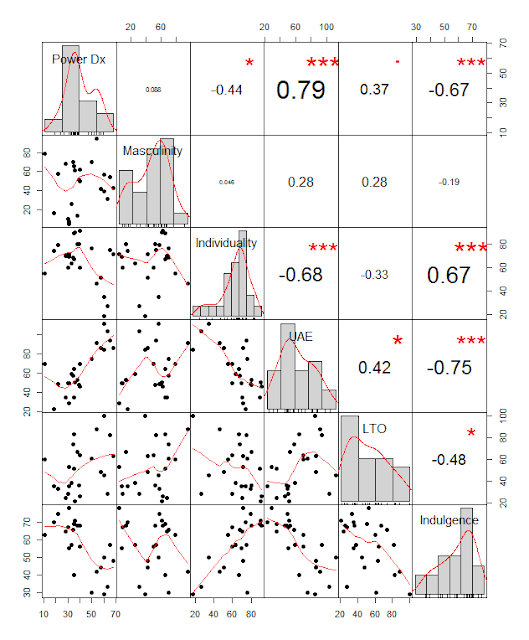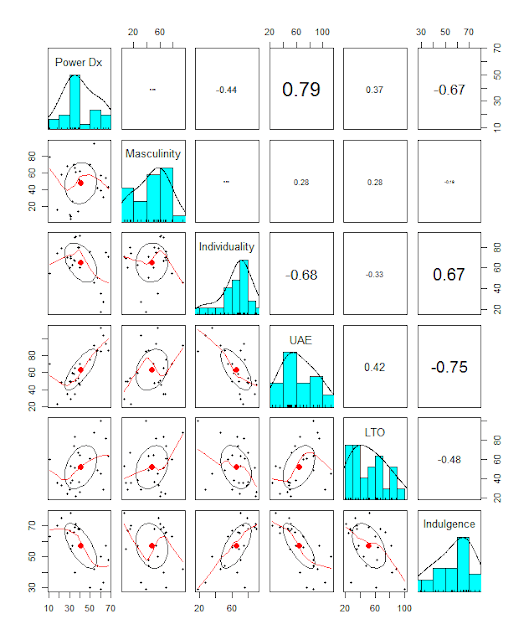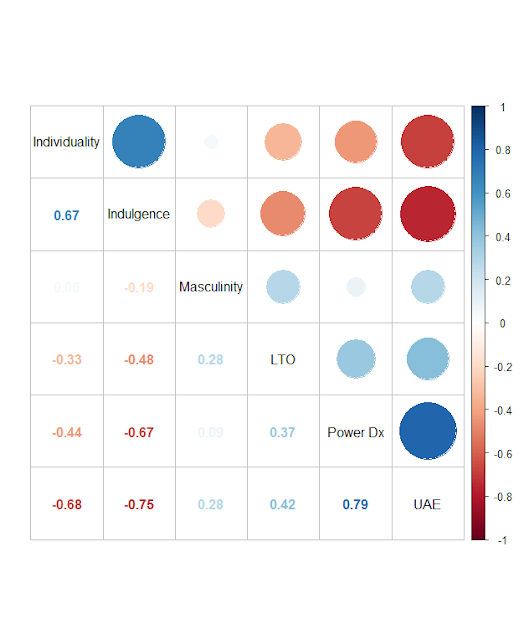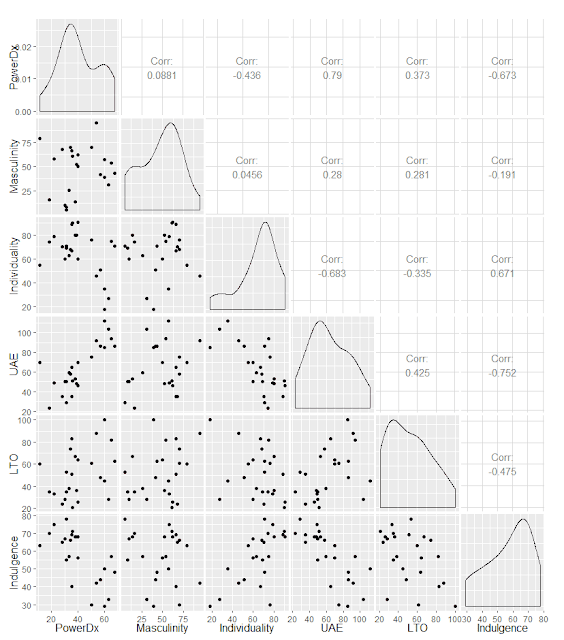### Charting Correlation Matrices in R

I noticed this very simple, very powerful article by James Marquez, Seven Easy Graphs to Visualize Correlation Matrices in R, in the Google+ community, R Programming for Data Analysis, so thought to give it a try, since I started some of my current analyses a decade ago by generating correlation matrices in Excel, which I've sometimes redone and improved in R.

Some of these packages are only designed for display, or as extensions to ggplot2:
These two are focused on more complex analysis:
As for data, I used Hofstede's culture dimensions, limited to developed countries. Using a broader and larger set of of countries would significantly reduce the correlations, in that only individuality and power distance would show a strong relationship, negative. The source data is here.

``````
# Charting correlations in R
# Source: http://jamesmarquezportfolio.com/correlation_matrices_in_r.html

# Clear workspace
rm(list = ls())

# Set working directory
setwd("../Data")
getwd()

oecdData <- read.table("OECD - Quality of Life.csv", header = TRUE, sep = ",")
hofsted.vectors <- oecdData[,c('HofstederPowerDx', 'HofstederMasculinity', 'HofstederIndividuality', 'HofstederUncertaintyAvoidance', 'HofstederLongtermOrientation', 'HofstederIndulgence')]

#rename columns
names(hofsted.vectors)[1:6] = c('PowerDx', 'Masculinity', 'Individuality', 'UAE', 'LTO', 'Indulgence')

# PerformanceAnalytics
install.packages("PerformanceAnalytics", dependencies = TRUE)
library("PerformanceAnalytics")
chart.Correlation(hofsted.vectors, histogram = TRUE, pch = 19)# psych
install.packages("psych", dependencies = TRUE)
library(psych)
pairs.panels(hofsted.vectors, scale = TRUE)# corrplot
install.packages("corrplot", dependencies = TRUE)
library(corrplot)
corrplot.mixed(cor(hofsted.vectors), order = "hclust", tl.col = "black")# GGally
install.packages("GGally", dependencies = TRUE)
library(GGally)
ggpairs(hofsted.vectors)# ggcorrplot
install.packages("ggcorrplot", dependencies = TRUE)
library(ggcorrplot)
ggcorrplot(cor(hofsted.vectors), p.mat = cor_pmat(hofsted.vectors), hc.order = TRUE, type = 'lower')``````